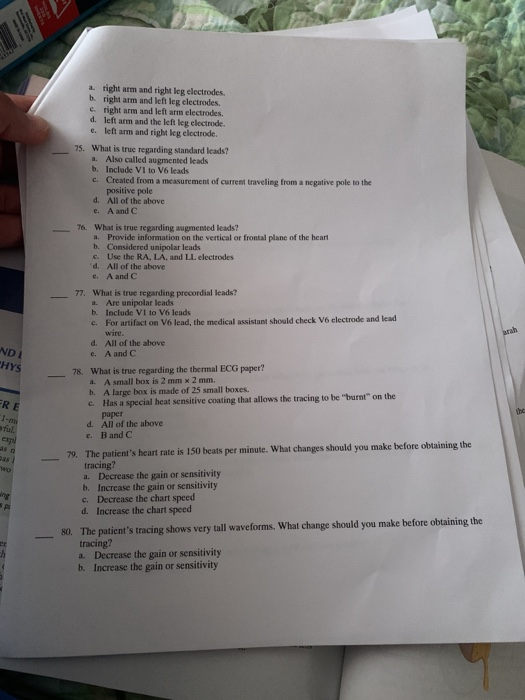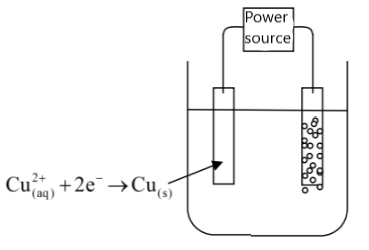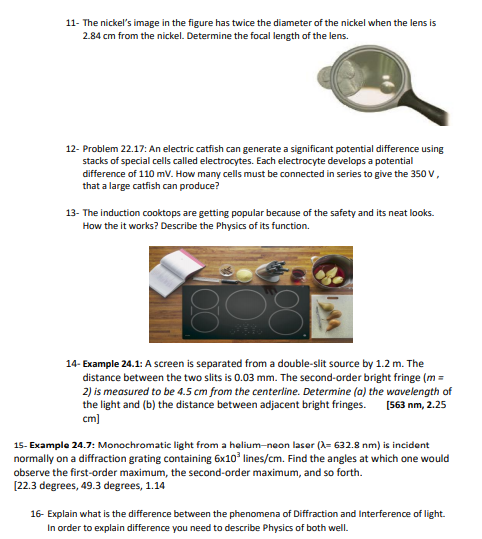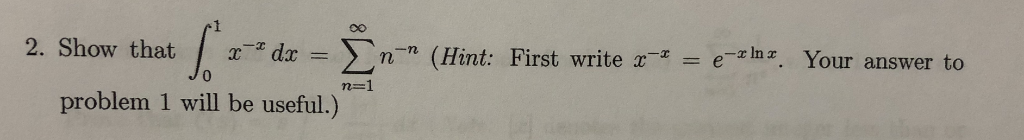# An electrolytic cellcontains a pair of inert metal electrodes in a solution containing 1.00 M nickel(II) sulfate at pH = 0.00. Hint: Recall that pH = -log10[H+]. The pH is increased gradually until th... related homework questions

• #### An electrolytic cellcontains a pair of inert metal electrodes in a solution containing 1.00 M nickel(II) sulfate at pH = 0.00. Hint: Recall that pH = -log10[H+]. The pH is increased gradually until th...

An electrolytic cellcontains a pair of inert metal electrodes in a solution containing 1.00 M nickel(II) sulfate at pH = 0.00. Hint: Recall that pH = -log10[H+]. The pH is increased gradually until the electrolysis product changes. What is the new product and at what pH does this occur?

• #### It’s review question, I need this as soon as possible. Thank you 3) For thè diferential equation: (a) The point zo =-1 is an ordinary point. Compute the recursion formula for the coefficients of...It’s review question, I need this as soon as possible. Thank you 3) For thè diferential equation: (a) The point zo =-1 is an ordinary point. Compute the recursion formula for the coefficients of the power series solution centered at zo- -1 and use it to compute the first three nonzero terms of the power series when -1)-s and v(-1)-0....

• #### Dont copié formé thé book oh ya dont copié formé thé book cause you Oiil inde up being triste soi remembré not toi copié frome thé book oh ya

Dont copié formé thé book oh ya dont copié formé thé book cause you Oiil inde up being triste soi remembré not toi copié frome thé book oh ya!translation in english please!

• #### DSuppose \$39oo is deposited in a savings account that increases exponentially.Detamine thě APv if the acount...DSuppose \$39oo is deposited in a savings account that increases exponentially.Detamine thě APv if the acount increases to \$t020 in 4 years. Ass ume tne interest Vale remains Constant and no additional deposits or Withdrawals are made. (a.) Let pbe the APY. Note tnat if tme inital balaqe is yo, ne year later tne balane is %more. P- 3 (Tpe...

• #### a. right arm and right leg electrodes. b. right arm and left leg electrodes. e right arm and left arm electrodes. d...a. right arm and right leg electrodes. b. right arm and left leg electrodes. e right arm and left arm electrodes. d. left arm and the left leg electrode. e left arm and right leg electrode. 75. What is true regarding standard leads? Also called augmented leads b. Include VI to V6 leads c. Created from a measurement of current...

• #### If a nickel contains 3.0 gram of copper and 1.2 gram of nickel How many kilograms of copper must be combines with 500 kilograms of nickel to make nickel coins

If a nickel contains 3.0 gram of copper and 1.2 gram of nickel How many kilograms of copper must be combines with 500 kilograms of nickel to make nickel coins?

• #### If a nickel contains 3.9 gram of copper and 1.2 gram of nickel How many kilograms of copper must be combines with 500 kilograms of nickel to make nickel coins

If a nickel contains 3.9 gram of copper and 1.2 gram of nickel How many kilograms of copper must be combines with 500 kilograms of nickel to make nickel coins?

• #### if a reaction between nickel (II) sulfate an d sodium hydroxide produces nickel (II) hydroxide and sodium sulfate and 4.4 moles of NaOH are used how many moles of the other reactants or products are used or produced

if a reaction between nickel (II) sulfate an d sodium hydroxide produces nickel (II) hydroxide and sodium sulfate and 4.4 moles of NaOH are used how many moles of the other reactants or products are used or produced? How do I set these problems up?

• #### Given the following electrolytic cell: The current is discharged into the electrolytic cell containing the solution CuSO4(aq) 1.0M at 25 oC. During the operation of the cell, copper Cu(s) is deposite...Given the following electrolytic cell: The current is discharged into the electrolytic cell containing the solution CuSO4(aq) 1.0M at 25 oC. During the operation of the cell, copper Cu(s) is deposited on one electrode and oxygen O2(g) gas is released, near the second electrode. O2(g) + 4H3O+(aq) + 4e- 6H2O(l) Eo= 1.23V Cu2+(aq) + 2e- Cu(s)    Eo= 0.34V A. Write...

• #### Given the following electrolytic cell: The current is discharged into the electrolytic cell containing the solution CuS...Given the following electrolytic cell: The current is discharged into the electrolytic cell containing the solution CuSO4(aq) 1.0M at 25 oC. During the operation of the cell, copper Cu(s) is deposited on one electrode and oxygen O2(g) gas is released, near the second electrode. O2(g) + 4H3O+(aq) + 4e- 6H2O(l) Eo= 1.23V Cu2+(aq) + 2e- Cu(s)    Eo= 0.34V A. The...

• #### which 3 are correct a) if x= -10^4 then log10 x = -4 b)if x= 2^8 then log 2x = 8 c) log2 2= 4 d) if x= 3 then log10 3=x e) log 10 256-2log 10 16=0 f)log 10 (a-b)= log 10 a/log 10 b g) the gradient of the graph of y= 2e^x at x= 2 is 2e^2 h) the gr

which 3 are correct a) if x= -10^4 then log10 x = -4 b)if x= 2^8 then log 2x = 8 c) log2 2= 4 d) if x= 3 then log10 3=x e) log 10 256-2log 10 16=0 f)log 10 (a-b)= log 10 a/log 10 b g) the gradient of the graph of y= 2e^x at x= 2 is 2e^2...

• #### Solve log10(8 x) − log10(1 + √x) = 2 for x

Solvelog10(8 x) − log10(1 + √x) = 2for x.

• #### I have the answers but need to see these worked out. Thanks! 11- The nickel's image in the figure has twice the diameter of the nickel when the lens is 2.84 cm from the nickel. Determine the foca...I have the answers but need to see these worked out. Thanks! 11- The nickel's image in the figure has twice the diameter of the nickel when the lens is 2.84 cm from the nickel. Determine the focal length of the lens. 12- Problem 22.17: An electric catfish can generate a significant potential difference using stacks of special cells called...

• #### 2.08 grams of anhydrous barium chloride was dissolved in water to make 50.00mL of solution and then added to 50.00mL of an aqueous solution containing 2.84 g of anhydrous sodium sulfate. A white precipitate of barium sulfate formed. How do you...?

a) Calculate the mass (in grams) of the precipitate that was formed b) Calculate the concentration (in mol/L) of sulfate ions in the final solution

• #### #0.98 g# of the metal sulfate was dissolved in water and excess of barium chloride was chloride was added. The precipitate barium sulfate weighed #0.95 g#. Calculate the equivalent weight of metal?

Also, write the reaction involved.

• #### 2. Show thatf-dr= nn (Hint: First write a n-n (Hint: First Your answer to 0 problem 1 will be useful 2. Show thatf-dr= nn (Hint: First write a n-n (Hint: First Your answer to 0 problem 1 will be...2. Show thatf-dr= nn (Hint: First write a n-n (Hint: First Your answer to 0 problem 1 will be useful 2. Show thatf-dr= nn (Hint: First write a n-n (Hint: First Your answer to 0 problem 1 will be useful

• #### A 21.5 g sample of nickel was treated with excess silver nitrate solution to produce silver metal and nickel(II) nitrate...

A 21.5 g sample of nickel was treated with excess silver nitrate solution to produce silver metal and nickel(II) nitrate. The reaction was stopped before all the nickel reacted, and 36.5 g of solid metal (nickel and silver) is present. Calculate the mass of solid silver metal present.

• #### Ksp=2.4x10^-5 for calcium sulfate. What is the molar solubility of calcium sulfate in pure water? What is the mass solubility of calcium sulfate in pure water, expressed in g/L?

Ksp=2.4x10^-5 for calcium sulfate. What is the molar solubility of calcium sulfate in pure water? What is the mass solubility of calcium sulfate in pure water, expressed in g/L?

• #### Two 4.0-cm-diameter aluminum electrodes are spaced 0.50 mm apart. The electrodes are connected to...

Two 4.0-cm-diameter aluminum electrodes are spaced 0.50 mm apart. The electrodes are connected to a 100 V battery. a.) What is the capacitance? b.) What is the magnitude of the charge on each electrode?

Need Online Homework Help?